# Test Prep Plan - Take a practice test

Take this practice test to check your existing knowledge of the course material. We'll review your answers and create a Test Prep Plan for you based on your results.
How Test Prep Plans work
1
2Based on your results, we'll create a customized Test Prep Plan just for you!
3Study smarter
Study more effectively: skip concepts you already know and focus on what you still need to learn.

# FTCE Math: Properties of Triangles Chapter Exam

Exam Instructions:

Choose your answers to the questions and click 'Next' to see the next set of questions. You can skip questions if you would like and come back to them later with the yellow "Go To First Skipped Question" button. When you have completed the practice exam, a green submit button will appear. Click it to see your results. Good luck!

### Page 1

#### Question 3 3. If angle a is 66 degrees and angle b is 54 degrees, what is the measure of angle d?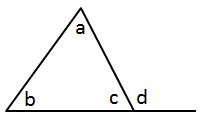#### Question 4 4. If segment BG has a length of 24 units, and G is the centroid of the triangle, how long is segment BE?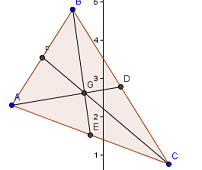#### Question 5 5. What type of triangle is pictured?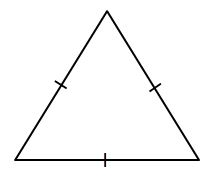### Page 2

#### Question 7 7. What type of triangle is pictured?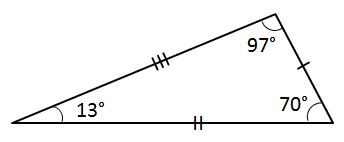#### Question 8 8. If angle a is 68 degrees and angle b is 37 degrees, what is the measure of angle c?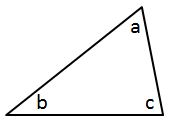#### Question 9 9. Given the triangle PQE, calculate the measure of Angle Q.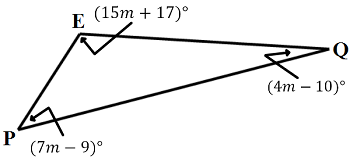#### Question 10 10. If angle a is 60 degrees, what is the measure of angle b?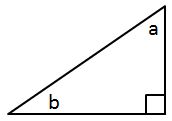### Page 3

#### Question 11 11. In the pictured triangle, identify the altitude(s).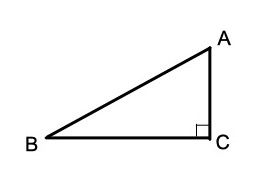#### Question 12 12. What type of triangle is pictured?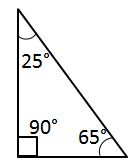#### Question 13 13. Find the orthocenter of triangle ABC with A ( 2 , 0 ) , B ( 2 , 4 ) , and C ( 5 , 0 ).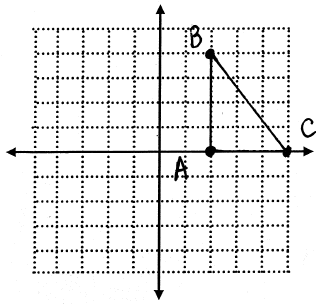#### Question 14 14. In triangle GBN, Angle G is seven more than three times a number, Angle B is five more than four times the number, and Angle N is twelve less than five times the number. Solve for the measure of Angle G.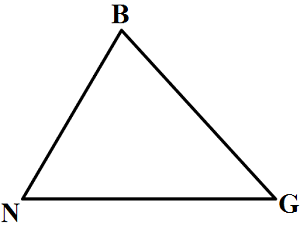#### Question 15 15. If angle a is 80 degrees, what is the measure of angle d?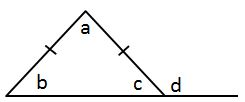### Page 4

#### Question 17 17. Given the triangle ABC, solve for the value of y.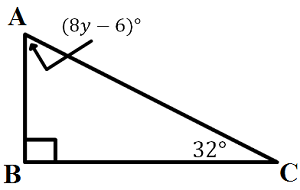#### Question 18 18. What type of line segment is represented by the dotted line in the pictured triangle?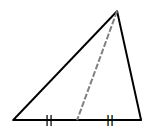### Page 5

#### Question 21 21. In the figure below, Angle 13 = 10y and Angle 11 = 3y + 11. Determine the measure of Angle 16.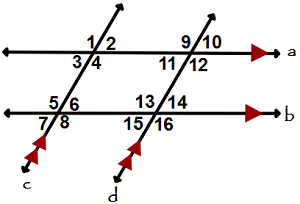#### Question 22 22. In the figure below, Angle 7 = 15x and Angle 10 = 9x + 30. Determine the measure of Angle 10.#### Question 23 23. If angle a is 30 degrees and angle d is 70 degrees, what is the measure of angle b?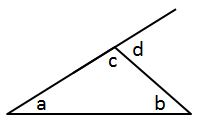#### Question 25 25. What type of triangle is pictured?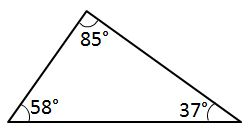### Page 6

#### Question 30 30. In the pictured triangle, which of the following lines is an altitude?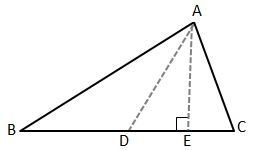#### FTCE Math: Properties of Triangles Chapter Exam Instructions

Choose your answers to the questions and click 'Next' to see the next set of questions. You can skip questions if you would like and come back to them later with the yellow "Go To First Skipped Question" button. When you have completed the practice exam, a green submit button will appear. Click it to see your results. Good luck!

Support